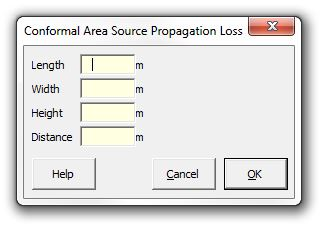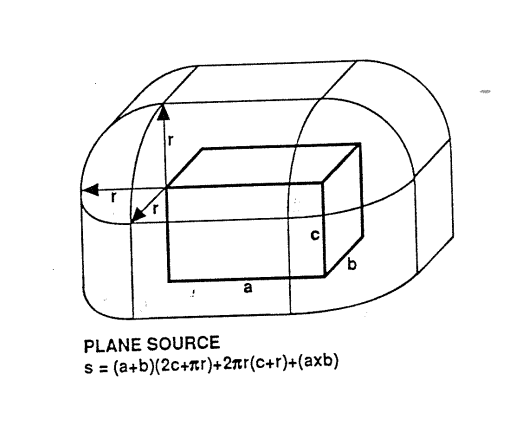﻿ Conformal Area### Strutt Help

Conformal Area Source Propagation Loss    1/1, 1/3

Strutt|Propagation|Conformal Area provides the correction for a conformal area, given the length, width, height and distance in metres from the source.The image below illustrates that a conformal area has rounded corners, which will result in the conformal area calculation becoming equivalent to a point source calculation for large distances.This is implemented as:

x = 10log_10(S), where

S=(A+B)(2C+pi r)+2 pi r(C+r)+(AB)

Note that this calculation assumes the source is located on a reflective surface; at large dimensions the results from this calculation converge to the same as hemispherical distance attenuation: Spherical Correction.

References:

• Arup Electrical Services Guide (red book) - source of the Conformal Area image above